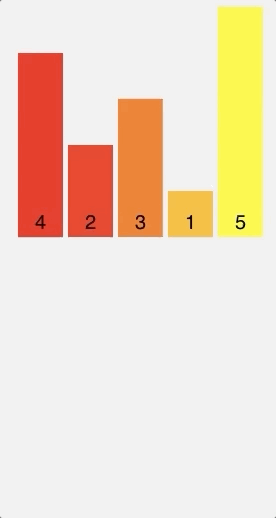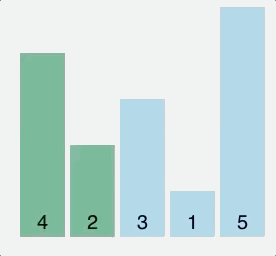# Sorting Algorithms with Javascript (Part 1)

##### Oct 18, 2018

I’ve been learning a lot about data structures and algorithms lately and I’ve noticed in my reading that there aren’t a lot of examples showing implementations of algorithms in Javascript. You’ll mostly find examples in Java, Python, C, C++ etc. Maybe there’s a reason for preferring these languages over Javascript? I’m not sure.

In this first part, I’m going to show Javascript implementations of three sorting algorithms:

• Merge sort
• Insertion sort
• Bubble sort

This is not intended to be an in-depth explanation on the ins and outs of how the algorithms work and their performance. If you’d rather read about that, here’s a nice resource I found: Sorting Algorithms

To keep things simple, I’ll be sorting a simple list `list` having only 5 elements `[4, 2, 3, 1, 5]`.

## Merge Sort

Merge sort uses a divide-and-conquer approach to sort elements in an array. Basically, what this means is that instead of dealing with the array as a whole, it continually splits it in half until both halves are sorted, then the halves are merged into one solved list.

#### Visual#### Code

``````function mergeSort(list) {
const len = list.length
// an array of length == 1 is technically a sorted list
if (len == 1) {
return list
}

// get mid item
const middleIndex = Math.ceil(len / 2)

// split current list into two: left and right list
let leftList = list.slice(0, middleIndex)
let rightList = list.slice(middleIndex, len)

leftList = mergeSort(leftList)
rightList = mergeSort(rightList)

return merge(leftList, rightList)
}

// Solve the sub-problems and merge them together
function merge(leftList, rightList) {
const sorted = []
while (leftList.length > 0 && rightList.length > 0) {
const leftItem = leftList
const rightItem = rightList
if (leftItem > rightItem) {
sorted.push(rightItem)
rightList.shift()
} else {
sorted.push(leftItem);
leftList.shift()
}
}

// if left list has items, add what is left to the results
while (leftList.length !== 0) {
sorted.push(leftList)
leftList.shift()
}

// if right list has items, add what is left to the results
while (rightList.length !== 0) {
sorted.push(rightList)
rightList.shift()
}

// merge the left and right list
return sorted
}

const list = [4, 2, 3, 1, 5]

const sorted = mergeSort(list)

console.log(sorted)
``````

## Insertion Sort

Insertion sort builds the final sorted list one element at a time. It does this by taking one element, comparing it to the rest of elements in the list, finding its right position, and then placing it there.

This is known as comparison-based sorting.

#### Visual#### Code

``````function insertionSort(list) {
const len = list.length
for (let i = 1; i < len; i++)
{
if (list[i] < list)
{
// move current element to the first position
list.unshift(list.splice(i,1))
}
else if (list[i] > list[i-1])
{
// maintain element position
continue
}
else {
// find where element should go
for (let j = 1; j < i; j++) {
if (list[i] > list[j-1] && list[i] < list[j])
{
// move element
list.splice(j, 0, list.splice(i,1))
}
}
}
}
return list
}

const list = [4, 2, 3, 1, 5]

const sorted = insertionSort(list)

console.log(sorted)
``````

## Bubble Sort

Another example of a comparison-based sorting algorithm, Bubble sort compares each pair of elements in a list and swaps them if they are out of order until the list is sorted.

#### Visual#### Code

``````function bubbleSort(list)
{
let swapped
let n = list.length-1
do {
swapped = false
for (let i=0; i < n; i++)
{
// compare pairs of elements
// if left element > right element, swap
if (list[i] > list[i+1])
{
const temp = list[i]
list[i] = list[i+1]
list[i+1] = temp
swapped = true
}
}
}
// continue swapping until sorted
while (swapped)

return list
}

const list = [4, 2, 3, 1, 5]

const sorted = bubbleSort(list)

console.log(sorted)
``````

Thats it! And, incase you’re wondering, I used this site to make the visuals.

In the next part, I’ll be going through:

• Quick sort
• Heap sort
• Counting sort